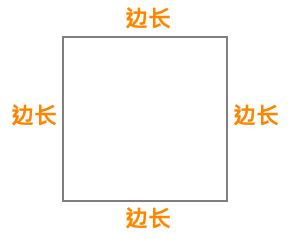# 正方形的面积正方形的面积 = 边长 x 边长

## 计算正方形的面积

 边长 正方形的面积

## 完美正方形

### 完美正方形的例子

1 = 1 x 1 = 12
4 = 2 x 2 = 22
9 = 3 x 3 = 32
16 = 4 x 4 = 42
25 = 5 x 5 = 52
36 =​​ 6 x 6 = 62
49 = 7 x 7 = 72
64 = 8 x 8 = 82
81 = 9 x 9 = 92
100 = 10 x 10 = 102
121 = 11 x 11 = 112
144 = 12 x 12 = 122
169 = 13 x 13 = 132
196 = 14 x 14 = 142
225 = 15 x 15 = 152
256 = 16 x 16 = 162
289 = 17 x 17 = 172
324 = 18 x 18 = 182
361 = 19 x 19 = 192
400 = 20 x 20 = 202
441 = 21 x 21 = 212
484 = 22 x 22 = 222
529 = 23 x 23 = 232
576 = 24 x 24 = 242
625 = 25 x 25 = 252